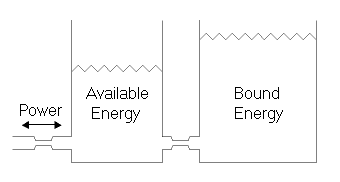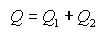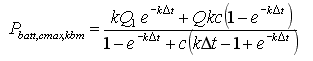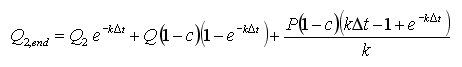# HOMER Grid 1.2

 Navigation: HOMER's Calculations Kinetic Battery ModelHOMER uses the Kinetic Battery Model (Manwell and McGowan, 1993) to determine the amount of energy that can be absorbed by or withdrawn from the storage bank each time step. The Kinetic Battery model is a two-tank model with kinetics that match lead acid battery behavior. The first tank contains "available energy," or energy that is readily available for conversion to DC electricity. The second tank contains "bound energy," or energy that is chemically bound and therefore not immediately available for withdrawal. The following diagram illustrates the concept.Three parameters are used to describe this two tank system. The maximum (or theoretical) storage capacity (Qmax) is the total amount of energy the two tanks can contain. The capacity ratio (c) is the ratio of the size of the available energy tank to the combined size of both tanks. The rate constant (k) relates to the conductance between the two tanks, and is therefore a measure of how quickly the storage can convert bound energy to available energy or vice versa. HOMER determines these three parameters from the storage's capacity curve, which you specify in the Storage library.

The total amount of energy stored in the Storage Component at any time is the sum of the available and bound energy, hence:where Q1 is the available energy and Q2 is the bound energy.

Using differential equations, one can show that the maximum amount of power that the Storage Component can discharge over a specific length of time (Δt) is given by the following equation:Similarly, the maximum amount of power that the storage can absorb over a specific length of time is given by the following equation:The preceding two equations give the allowable range for the power into or out of the storage bank in any one time step. (HOMER imposes two additional limitations on the charge power. For more information, see the section on calculating the maximum charge power. After HOMER calculates the actual charge or discharge power, it calculates the resulting amount of available and bound energy at the end of the time step using the following two equations:where Q1 = the available energy [kWh] at the beginning of the time step Q2 = the bound energy [kWh] at the beginning of the time step Q1,end = the available energy [kWh] at the end of the time step Q2,end = the bound energy [kWh] at the end of the time step, P = the power [kW] into (positive) or out of (negative) the storage bank Δt = the length of the time step [h]